# DAV Class 7 Maths Chapter 8 Brain Teasers Solutions

The DAV Maths Book Class 7 Solutions Pdf and DAV Class 7 Maths Chapter 8 Brain Teasers Solutions of Triangle and Its Properties offer comprehensive answers to textbook questions.

## DAV Class 7 Maths Ch 8 Brain Teasers Solutions

Question 1.
A. Tick (✓) the correct option:
(i) In ΔABC, AB + BC > __________
(a) AB
(b) BC + AC
(c) AC
(d) NoneGiven: A ΔABC having side AB, BC and CA.
Now, in ΔABC, AB + BC > AC [∵ The sum of the lengths of any two sides of a triangle is greater than the length of third side.]
Hence, (c) is the correct option. B

(ii) The centroid of a triangle divides each median in the ratio:
(a) 2:1
(b) 2:3
(c) 3:1
(d) 1:3
The centroid of a triangle divides each one of the medians in the ratio 2:1.
Hence, (a) is the correct option.

(iii) The hypotenuse of a right triangle is 17 cm and one of the side is 8 cm. The other side is
(a) 16 cm
(b) 12 cm
(c) 13 cm
(d) 15 cm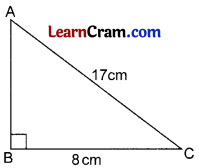Let in AABC, BC = 8 cm and AC = 17 cm.
∴ By Pythagoras theorem,
(AB)2 + (BC)2 = (AC)2
⇒ (AB)2 + (8)2 = (17)2
⇒ (AB)2 + 64 = 289
⇒ (AB)2 = 289 – 64
⇒ (AB)2 = 225
⇒ AB = 15 cm
So, the other side of the right triangle is 15cm.
Hence, (d) is the correct answer.

(iv) The point of concurrence of the altitudes of a triangle is called
(a) Orthocentre
(b) Circumcentre
(c) Centroid
(d) Incentre
The point concurrence of the altitudes of a triangle is called the orthocentre of the triangle.
Hence, (a) is the correct option.

(v) In ΔPQR, if ΔPRS is an exterior angle at R, then the interior adjacent angle at R is —
(a) ∠RQP
(b) ∠QPR
(c) ∠PRQ
(d) ∠PQR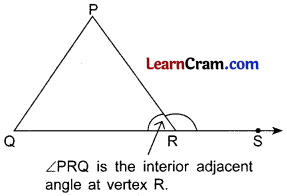∠PRS is the exterior angle of ΔPQR at point R and ∠PRQ is the adjacent angle at vertex R.
Hence, (c) is the correct answer.(i) What is the point of concurrence of angle bisector of a triangle called? Where does it lie?
The point of concurrence of the angle bisectors of a triangle is called the incentre of the triangle.
Since the angle bisectors of a triangle will all lie in the interior of the triangle, the incentre of a triangle always lies in its interior.

(ii) The vertical angle of an isosceles triangle is 80°. Find measurement of its base angles.
Let ΔABC be an isosceles triangle with AB = BC.
⇒ ∠B = ∠C
[∵ Angles opposite to equal sides of a triangle are equal]
Now, by angle sum property, we have
∠A + ∠B + ∠C = 180°
80° + ∠B + ∠C = 180° [∵ ∠A = 80° (gIVEN)]
80° + ∠B + ∠B = 180°
80° + 2∠B = 180°
2∠B = 180° – 80°
2∠B = 100°
2∠B = $$\frac{100^{\circ}}{2}$$ = 50°
But ∠B = ∠C
∠C = 50°
Hence, the base angles are ∠B = ∠C = 50°.

(iii) Find the value of x and y in the given figure.
We have, ∠ACD = ∠BAC + ∠ABC
[∵ In a triangle, the exterior angle is equal to the sum of the two interior opposite angles.]
⇒ 120° = x + 50°
⇒ 120° – 50° = x
⇒ 70° = x
⇒ x = 70°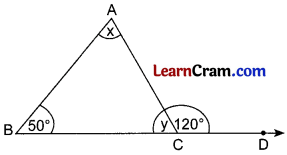Now, in ΔABC, we have
∠A + ∠B + ∠C = 180°
⇒ x + 50° + y = 180°
⇒ 70° + 50° + y = 180°
⇒ 120° + y = 180°
⇒ y = 180° – 120°
⇒ y = 60°
Hence, the values of x and y are 70° and 60° respectively.

(iv) Can the line segments 1.5 cm, 2 cm, 2.5 cm be the sides of a right angled triangle?
Since 1.5 + 2 > 2.5, 2 + 2.5 > 1.5, 2.5 + 1.5 > 2.
So, 1.5 cm, 2 cm, 2.5 cm can be the sides of a triangle.
Also, (1.5)2 + (2)2 = (2.5)2
⇒ 2.25 + 4 = 6.25
⇒ 6.25 = 6.25, which is true.
So, the square of one side of a triangle is equal to the sum of the squares of the other two sides, then it is a right angled triangle with the angle opposite to the side 2.5 cm as a right angle.

(v) Where does the circumcentre of a right angled triangle lie?
In a right angled triangle, the circumcentre is at the mid-point of the hypotenuse, i.e., it lies on the triangle.
For example, in right angled ΔABC, the circumcentre X is at the mid-point of AC, i.e.; it lies on the ΔABC.

Question 2.
A man goes 5 m in east and then 12 m in north direction. Find the distance from the starting point.As per the figure given alongside
AC2 = AB2 + BC2 (Pythagoras Theorem)
= (5)2 + (12)2
= 25 + 144
= 169
∴ AC = $$\sqrt{169}$$ = 13 m
Hence the required distance = 13 m.

Question 3.
The width of a rectangle is 5 cm and its diagonal is 13 cm. What is its perimeter?
In right ΔABC,
AB2 + BC2 = AC2 (Pythagoras Theorem)
⇒ AB2 +(5)2 = (13)2
⇒ AB2 + 25 = 169
⇒ AB2 = 169 – 25
⇒ AB2 = 144
∴ AB = $$\sqrt{144}$$ = 12 cm
Perimeter = 2 (Length + Breadth) = 2(12 + 5) = 2 x 17 = 34 cm.Question 4.
In the given figure, ΔPQR and ΔSQR are isosceles,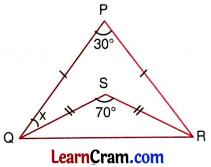(i) Find ∠PQR and ∠PRQ
(ii) Find ∠SQR and ∠SRQ
(iii) Find x
(i) PQ = PR (Given)
∠PQR = ∠PRQ (Angles opposite to equal sides)
∠PQR + ∠PRQ + ∠QPR = 180° (Angle sum property)
2∠PQR + 30 = 180°
2∠PQR = 180° – 30°
∠PQR = $$\frac{150}{2}$$ = 75°
∴ ∠PQR = ∠PRQ = 75°

(ii) In ΔSQR, SQ = SR
∴ ∠SQR = ∠SRQ
∠SQR + ∠SRQ + ∠QSR = 180°
2∠SQR + 70° = 180°
2∠SQR = 180° – 70°
2∠SQR = 110°
∠SQR = $$\frac{110}{2}$$ = 55°
Hence ∠SQR = ∠SRQ = 55°

(iii) x = ∠PQR – ∠SQR
= 75°- 55° = 20°

Question 4.
In given figure, ΔPQR is isosceles with PQ = PR . LM is parallel to QR with L on PQ and M on PR. Give reasons for each of the following statements:
(i) ∠Q = ∠R
(ii) ∠PLM = ∠Q
(iii) ∠PML = ∠R
(iv) ∠PLM = ∠PML
(v) ΔPLM is isosceles.
Solution:
(i) PQ = PR (given)
∠Q = ∠R (Angles opposite to equal sides)
(ii) ∠PLM = ∠Q (Corresponding angles are equal)
(iii) ∠PML = ∠R (Corresponding angles are equal)
(iv) ∠PLM = ∠PML [From (i), (ii) and (iii)]
(v) PQ = PR (given)
∴ ΔPQR is an isosceles triangle.

Question 5.
In an isosceles triangle, the base angle is twice as large as vertex angle. Find the angles of the triangle.
Solution:
Let the vertex angle be x
∴ Each of base angle = 2x
2x + 2x + x = 180° (Angle Sum Property of a triangle)
⇒ 5x = 180°
⇒ x = $$\frac{180^{\circ}}{5}$$ = 36°
Hence vertex angle is 36° and each of base angles is 36 × 2 = 72°.

Question 6.
A pole broke at a point but did not separate. Its top touched the ground at a distance of 5m from its base. If the point where it broke is at a height of 12 m from the ground, what was the total height of the pole before it broke?
Let AB be a pole and it broke at a point C and its top touches the ground at D such that BD = 5 m and BC = 12 mIn right triangle CBD,
CD2 = BD2 + BC2 (By Pythagoras Theorem)
= (5)2 + (12)2
= 25 + 144 = 169
CD = $$\sqrt{169}$$ = 13 m
Total height of the pole = BC + CD
= BC + AC
= 12 + 13
= 25 m
Hence the total height of the pole = 25 m.

Question 7.
∆ABC is right angled at C. Can you locate its orthocentre without drawing any altitude? If so, name it.
Right angle vertex is the orthocentre of the ΔABC see figure below.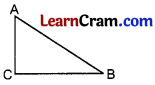Question 8.
Draw an equilateral triangle each of whose sides is 6 cm. Draw its medians. Are they equal?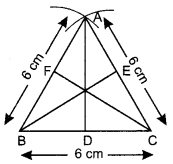(i) Draw A ABC with each side of 6 cm.
(ii) AD, BE and CF are the medians.
(iii) Medians AD = BE = CF = 5.2 cm (approx)

Question 9.
The exterior angle at C of a ΔABC is equal to 120°. Find the measure of all the angles of the triangle if ∠A = ∠B. What type of triangle is this?∠ACB + ∠ACD = 180° (Linear pair)
∠ACB + 120° = 180°
∠ACB = 180° – 120°
∠ACB = 60°
∠A = ∠B (given)
∠A + ∠B + ∠C = 180° (Angle Sum Property)
∠A + ∠A + 60° = 180°
2∠A = 180° – 60°
2∠A = 120°
∴ ∠A = $$\frac{120}{2}$$ = 60°
Hence ∠A = 60°, ∠B = 60° and ∠C = 60°.
ΔABC is an equilateral triangle.

Question 10.
In the given figure, find the measure of ∠1, ∠2, ∠3.∠3 + ∠PRS = 180° (Linear pair)
∠3 +136° = 180°
∠3 = 180° -136°
∠3 = 44°
∠2 + ∠TQR = 180° (Linear pair)
∠2 + 104° = 180°
∠2 = 180° – 104°
∠2 = 76°
∠1 + ∠2 + ∠3 = 180° (Angle Sum Property)
∠1 + 76° + 44° = 180°
∠1 + 120° = 180°
∠1 = 180° – 120° = 60°
Hence ∠1 = 60°, ∠2 = 76° and ∠3 = 44°.Question 11.
In the given figure, find the measure of ∠CAB, ∠ABC, ∠ACB.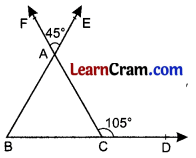∠ACB + ∠ACD = 180° (Linear pair)
∠ACB + 105° = 180°
∠ACB = 180° – 105° = 75°
∠EAF = ∠BAC (Vertically opposite angles)
∠BAC = 45°
∠ABC + ∠BCA + ∠CAB =180° (Angle Sum Property)
∠ABC + 75° + 45° = 180°
∠ABC + 120° = 180°
∠ABC = 180° -120° = 60°
Hence ∠CAB = 45°, ∠ABC = 60° and ∠ACB = 75°

Question 12.
Find x and y in the following figure.∠x = ∠CAB + ∠ABC (Exterior angle of a ΔABC)
∠x = 30° + 30°
∠x = 60°
∠y = ∠EDC + ∠ECD (Exterior angle of a ΔEDC)
∠y = 30° + 60°
∠y = 90°
Hence x = 60° and y = 90°.

Question 13.
The lengths of two sides of a triangle are 12 cm and 15 cm. Between what two measures should the length of the third side fall?
As the sum of any two sides of a triangle is always greater than the third side.
Length of third side may be greater than 15 -12 = 3 cm.
and less than 15 + 12 = 27 cm
Hence 3 < length of third side < 27 cm.

## DAV Class 7 Maths Chapter 8 HOTS

Question 1.
In the given figure, AE = AC, ∠BAC = 40°, ∠ACF = 75° and BCF is a line. Prove that BE = CE.Given: ΔAABC in which ∠BAC = 40°. BC is produced to F such that ∠ACF = 75°. Also, E is a point on AB such that AE = AC.
To Prove: BE = CE
Proof: In ΔAEC, AE = EC [Given]
∴ ∠AEC = ∠ACE [∵ Angles opposite to equal sides of a triangle are equal]
= ∠ ACE = 70°
Now, ∠A + ∠AEC + ∠ACE = 180°
⇒ 40° + ∠AEC + ∠ACE = 180°
⇒ 40° + ∠AEC + ∠AEC = 180°
⇒ 40° + 2∠AEC = 180°
⇒ 2∠AEC = 180° – 40°
⇒ 2∠AEC = 140°
⇒ ∠AEC = $$\frac{140^{\circ}}{2}$$ = 70°
Now, ∠ACF = ∠A + ∠B [∵ In a triangle, the exterior angle is equal to the sum of the two interior opposite angles]
⇒ 75 = 40° + ∠B
⇒ 75 – 40° = ∠B
⇒ 35 = ∠B
⇒ ∠B = 35°

As BCF is a straight line.
∴ ∠ECB + ∠ACE + ∠ACF = 180°
∠ECB + 70° + 75° = 180°
∠ECB + 145° = 180°
∠ECB = 180° – 145°
∠ECB = 35°
Since ∠EBC = ∠ECB = 35°
∴ BE = CE [∵ Sides opposite to equal angles of triangle are equal]
Hence, proved.

Question 2.
In the given figure, BO and CO are the bisectors of the exterior angles meeting each other at O. If ∠A = 70° find ∠BOC.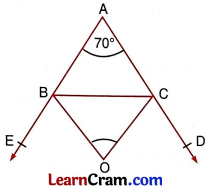In ΔABC, we have
∠A + ∠B + ∠C = 180° [Angle sum property of a triangle]
⇒ 70° + ∠B + ∠C = 180° [∵ ∠A = 70° (Given)]
⇒ ∠B + ∠C = 180° – 70°
⇒ ∠B + ∠C = 110°

Now, ∠CBE = ∠A + ∠ACB [∵ In a triangle, the exterior angle is equal to the sum of the two interior opposite angles]
⇒ ∠OBE + ∠OBC = ∠A + ∠ACB
⇒ ∠OBC + ∠OBC = ∠A + ∠ACB [. OB is the angle bisector of ¿CBEJ
⇒ 2∠OBC = ∠A + ∠ACB

Also, ∠BCD = ∠CBA + ∠A
⇒ ∠OCD + ∠OCB = ∠OEA + ∠A
⇒ ∠OCB + ∠OCB = ∠OEA + ∠A [: OC is the angle bisector of ∠BCD]
⇒ 2∠OCB = ∠OEA + ∠A …(2)

Adding equations (1) and (2), we get
⇒ 2∠OBC + 2∠OCB = ∠A + ∠ACB + ∠CBA + ∠A
⇒ 2(∠OBC + ∠OCB) = (∠A + ∠ACB + ∠CBA) + ∠A
⇒ 2(180° – ∠BOC) = 180° + 70° [Angle sum property of a triangle]
⇒ 2(180° – ∠BOC) = 250°
⇒ 180° – 125° = ∠BOC
⇒ 55° = ∠BOC
⇒ ∠BOC = 55°

## DAV Class 7 Maths Chapter 9 Enrichment Questions

Question 1.
In the given figure, AB = AC and BC = CD, find x, y, z.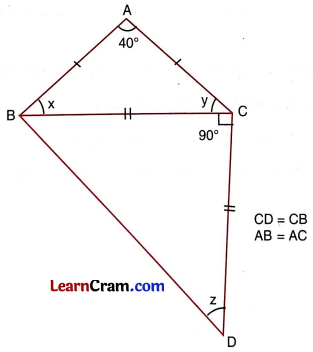In ΔABC, we have
AB = AC [Given]
∠B = ∠C [Angles opposite to equal sides of a triangle are equal]
x = y
Now, ∠A + ∠B + ∠C = 180°
⇒ 40° + x + y = 180°
⇒ 40° + x + x = 180°
⇒ 40° + 2x = 180°
⇒ 2x = 180 – 40°
⇒ 2x = 140°
⇒ x = $$\frac{140^{\circ}}{2}$$
⇒ x = 70

∴ x = y = 70°
Now, in ΔBCD, we have
CB = CD [Given]
∴ ∠CBD = ∠CBD [Angles opposite to equal sides of a triangle are equal]
⇒ ∠CBD = ∠CDB = z

Also, in ΔBCD,
⇒ ∠CBD + ∠BCD + ∠CDB = 180° [Angle sm property of a triangles]
⇒ z + 90 + z = 180° [∵ ∠CBD = ∠CDB = z]
⇒ 2z = 180° – 90°
⇒ 2z = 90°
⇒ z = $$\frac{90^{\circ}}{2}$$
⇒ z = 45°
Hence, x = y = 70° and z = 45°.

Question 2.
Two poles 15 m and 30 m high are standing straight on the ground. If their feet are 36 m apart, find the distance between their tops.
Suppose AB = 15 m and XY = 30 m are two poles standing straight on the ground.So, XY = XZ + ZY
⇒ 30 m = XZ + 15 m
⇒ XZ = 30m – 15 m
⇒ XZ = 15 m
Also, AZ = BY = 36 m

Now, in right angled ΔXZA
⇒ (AX)2 = (XZ)2 + (AZ)2 [By Pythagoras theorem]
⇒ (AX)2 = (15)2 + (36)2
⇒ (AX)2 = 225 + 1296
⇒ (AX)2 = 1521
⇒ AX = $$\sqrt{1521}$$ = 39 m
So, the distance between the tops of two poles is 39 m.

Question 1.
In an isosceles triangle ABC, AB = AC. ∠A = (2x + 15)°, ∠B = (3x + 20)° and ∠C = (x + 25)°, find the value of x and hence the measurement of the angles of ΔABC.
∠A + ∠B + ∠C = 180° (Angle Sum Property)
⇒ 2x + 15° + 3x + 20°+ x + 25°= 180°
⇒ 6x + 60° = 180°
∠A = (2x + 15)° = (2 × 20 + 15)° = 55°
∠B = (3x + 20)° = (3 × 20 + 20)° = 80°
∠C = (x + 25)° = (20 + 25) = 45°
Hence ∠A = 55°, ∠B = 80° and ∠C = 45°.Question 2.
The length of the hypotenuse of right triangle is 29 cm. If one of the remaining sides is 20cm long, find the length of the other side.
Let the other side be x cm
x2 + (20)2 = (29)2 (Pythagoras Theorem)
⇒ x2 + 400 = 841
⇒ x2 = 841 – 400
⇒ x2 = 441
⇒ x = $$\sqrt{441}$$
x = 21 cm
Hence the length of the required side = 21 cm.

Question 3.
In a right triangle right angled at C. AC = BC, prove that AB2 = 2AC2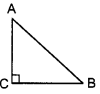In ΔABC, ∠C = 90° and AC = BC
∴ AB2 = AC2 + BC2 (Pythagoras theorem)
⇒ AB2 = AC2 + AC2 [v AC = BC]
⇒ AB2 = 2AC2
Hence proved.

Question 4.
Prove that the sum of all the angles of a quadrilateral is 360°.
Let ABCD is a quadrilateral. Join B and D to divide the quadrilateral in two triangles.In ΔABD,
∠A + ∠1 + ∠2 = 180° (Angle Sum Property)
In ΔBCD,
∠C + ∠3 + ∠4 = 180° (Angle Sum Property)
Add ∠A + ∠C + ∠1 + ∠3 + ∠2 + ∠4 = 360°
∠A + ∠C + ∠B + ∠D = 360°
⇒ ∠A + ∠B + ∠C + ∠D = 360°
Hence proved.

Question 5.
In an equilateral ΔABC, AD ⊥ BC. If each side of the triangle is 6 cm, find the length of AD.
AB = BC = AC = 6 cm
BD = $$\frac{1}{2}$$BC = $$\frac{1}{2}$$ × 6 = 3 cmAD2 = 36 – 9 = 27
.’. AD = $$\sqrt{27}=\sqrt{3 \times 3 \times 3}=3 \sqrt{3}$$ cm

Question 6.
In the given ΔPQR , ∠P = 40°. PQ = PR, find x and y.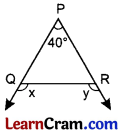PQ = PR (given)
∠Q = ∠R (Angles opposite to equal sides)
∠P = 40° (given)
∠Q + ∠R = 180° – 40° = 140°
∠Q = ∠R = $$\frac{140}{2}$$ = 70°
∠x + ∠Q = 180° (Linear pair)
∠x + 70° = 180°
∠x = 180°-70° = 110°
∠y + ∠R = 180° (Linear pair)
∠y + 70° = 180°
∠y = 180° – 70° = 110°
Hence ∠x = ∠y = 110°.Question 7.
O is any point interior to the ΔABC, prove that OA + OB + OC > $$\frac{1}{2}$$ (AB + BC + AC).
Sum of any two sides of a triangle is greater than the third side.
In ΔOBC,
OB + OC > BC ………(i)
In ΔOAC,
OA + OC > AC ………….(ii)
In ΔOAB,
OA + OB > AB ………….(iii)
Adding (i), (ii) and (iii) we get
20A + 20B + 20C > AB + BC + AC
2[OA +OB + OC] > AB + BC + AC
Hence OA + OB + OC > $$\frac{1}{2}$$ (AB + BC + AC).

Question 8.
Measurement of three sides of a triangle are given as under. In which case, the triangle is possible to construct?
(i) 8, 17, 50
8, 17, 50
Here, 8 + 17 = 25 < 50 ∴ Triangle is not possible to construct. (ii) 6,8,12 Answer: 6, 8, 12 Here, 6 + 8 = 14 > 12
∴ So, triangle is possible to construct.

(iii) 25, 26, 28
25, 26, 28
Here, 25 + 26 = 51 > 28
∴ So, triangle is possible to construct.

(iv) 8,17,50
8, 8, 7
Here, 8 + 7 = 15 > 8
∴ So, triangle is possible to construct.

Question 9.
State which of the following triplets are Pythagorean triplets?
(i) (8,15,17)
(8,15,17)
Here, (8)2 = 64, (15)2 = 225 and (17)2 = 289
(8)2 + (15)2 = (17)2 (Pythagoras theorem)
⇒ 64 + 225 = 289
⇒ 289 = 289
Hence, it is Pythagorean triplet.

(ii) (20,21,25)
(20,21,25)
Here, (20)2 = 400, (21)2 = 441, (25)2 = 625
(20)2 + (21)2 = (25)2 (Pythagoras theorem)
⇒ 400 + 441 ≠ 625
⇒ 841 ≠ 625
Hence it is not a Pythagorean triplet.

(iii) (6,8,10)
(6,8,10)
Here, (6)2 = 36, (8)2 = 64, (10)2 = 100
(6)2 + (8)2 = (10)2 (Pythagoras theorem)
⇒ 36 + 64 = 100
⇒ 100 = 100
Hence it is a Pythagorean triplet.

(iv) (5,12,13)
(5,12,13)
Here, (5)2 = 25, (12)2 = 144, (13)2 = 169
(5)2 + (12)2 = (13)2 (Pythagoras theorem)
⇒ 25 + 144 = 169
⇒ 169 = 169
Hence it is a Pythagorean triplet.Question 10.
Fill in the blanks.
(i) The point of intersection of the medians of a triangle is called __________.
Centroid

(ii) The point of intersection of the altitudes drawn from the vertices of a triangle to its opposite sides is called __________
Orthocentre

(iii) Orthocentre is the point of intersection of the __________ of a triangle.
altitudes

(iv) In a right triangle __________ is the largest side.
hypotenuse

(v) __________ of a triangle always lies inside the triangle.
Incentre

(vi) In an equilateral triangle, the three medians are __________ to each other.
Equal

(vii) Centroid of a triangle divides each median in the ratio __________